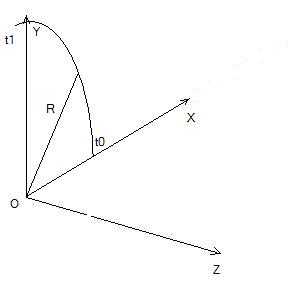# Circular Curve

## Detailed Description

group a3d_crvcircle

Entity type is kA3DTypeCrvCircle.

Version

2.0

The canonical form is defined with an A3DMiscCartesianTransformationData, its origin being the center of the circle and its vector X being the axis corresponding to zero parameter. The implicit parameterization is an angle expressed as radians in the range [0, 2PI].

A3DParameterizationData lets you specify reparameterization and trim.

Here are parameterization examples:

• To specify the interval in radians, set `m_dCoeffA` to 1.0 and `m_dCoeffB` to 0.0. These parameter values specify an identity conversion.

• To specify the interval in degrees, set `m_dCoeffA` to PI/180 and `m_dCoeffB` to 0.0. `m_dCoeffA` is the ratio of radians to degrees.

In the above example, the circular arc is in the XY plane (and therefore has an identity transformation), has radius R, and is restricted to the [ t0 , t1 ] interval.Example of a circular arc

If `m_dCoeffA` is 1.0 and `m_dCoeffB` is 0.0 and if the interval is defined as `t0=0` and `t1=PI/2`, then `t0` corresponds to the Cartesian coordinates (R,0,0) and `t1` to (0,R,0). These values for the `m_dCoeffA` and `m_dCoeffB` co-efficients indicate parameterization is in radians and starts at the X axis.

Note

Radius must be greater than 0.

Warning

Radius as a parameter can be modified by A3DMiscCartesianTransformationData when the transformation defines uniform scaling.

## Function Documentation

A3DStatus A3DCrvCircleGet(const A3DCrvCircle *pCrv, A3DCrvCircleData *pData)

Populates the A3DCrvCircleData structure.

Return values
• A3D_INITIALIZE_NOT_CALLED

• A3D_INVALID_DATA_STRUCT_SIZE

• A3D_INVALID_DATA_STRUCT_NULL

• A3D_INVALID_ENTITY_NULL

• A3D_INVALID_ENTITY_TYPE

• A3D_CRV_CANNOT_ACCESS_CANONICAL

• A3D_SUCCESS

Returns

A3D_SUCCESS in case of success or an error code

A3DStatus A3DCrvCircleCreate(const A3DCrvCircleData *pData, A3DCrvCircle **ppCrv)

Creates an A3DCrvCircle from A3DCrvCircleData structure.

Version

2.0

Return values
• A3D_INVALID_DATA_STRUCT_SIZE

• A3D_INVALID_DATA_STRUCT_NULL

• A3D_INTERVAL_INCONSISTENT_DATA

• A3D_SUCCESS

Returns

A3D_SUCCESS in case of success or an error code